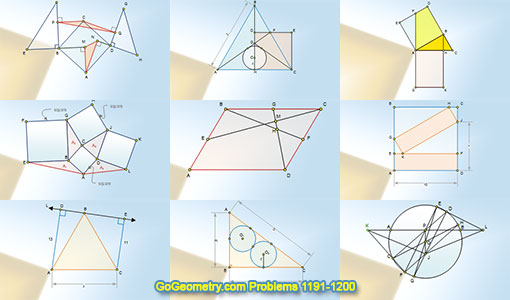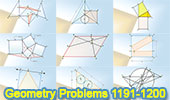# Geometry Problems 1191-1200. Level: High School, CollegeGeometry Problems 1191 - 1200Geometry Problem 1191 Circle, Chords, Diameter, Congruence, Midpoint, Collinearity, Bisector. Geometry Problem 1192 Trapezoid, Triangle, Diagonal, Midpoint, Area, Parallel. Geometry Problem 1193 3-4-5 Right Triangle, Congruent Circles, Tangent, Radius.Online Education, School, College Geometry Problem 1194 Equilateral Triangle, Perpendicular, Distance, Side, Metric Relations. Geometry Problem 1195 Square, Two Rectangles, Congruence, Metric Relations. Geometry Problem 1196 Parallelogram, Midpoint, Metric Relations. Geometry Problem 1197 Four Squares, Four Triangles, Equal Areas. Geometry Problem 1198 Right Triangle, Square, Rectangle, Quadrilateral, Altitude, Equal Areas. Geometry Problem 1199 Equilateral Triangle, Square, Altitude, Circle, Incircle, Inradius, Metric Relations. Geometry Problem 1200 Square, Right Triangle, Perpendicular, 90 Degrees, Equal Areas.

Home | Sitemap | Search | Geometry | Problems | Visual Index | 10 ProblemsEmail | Post a comment | by Antonio Gutierrez
Last updated: Apr 2, 2016